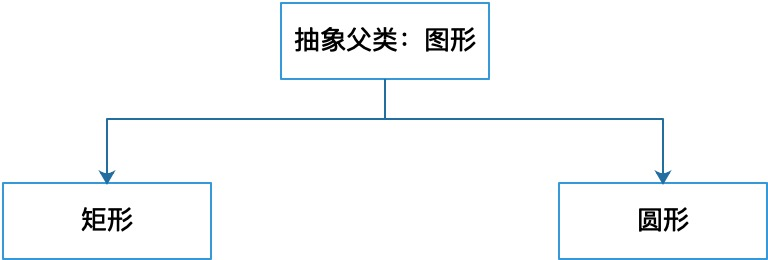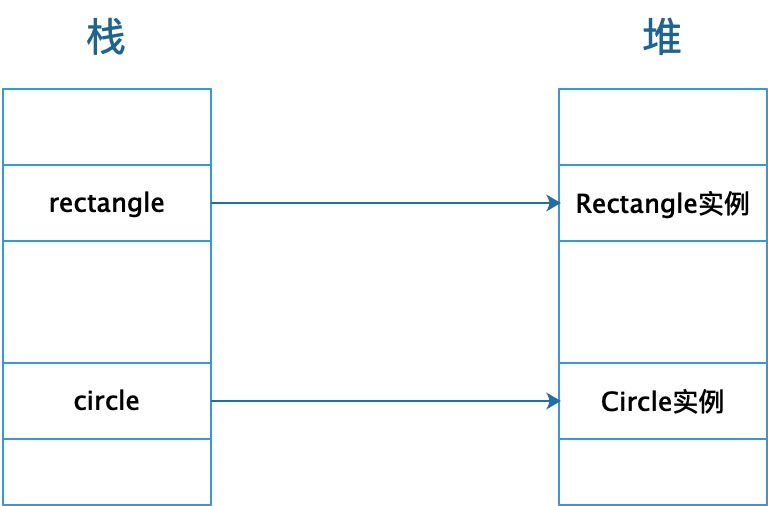# Java面向对象编程三大特征 - 多态

• 对于文章中出现的任何错误请大家批评指出，一定及时修改。
• 有任何想要讨论和学习的问题可联系我：zhuyc@vip.163.com。
• 发布文章的风格因专栏而异，均自成体系，不足之处请大家指正。

# Java面向对象编程三大特征 - 多态

## 一、抽象类### 1. 抽象与抽象类

• abstract可以修饰方法，修饰后被称为抽象方法
• abstract可以修饰类，修饰后被称为抽象类
• abstract不能与static修饰符同时使用
• abstract不能与final修饰符同时使用

• 抽象方法只能存在于抽象类中（接口在另外的文章中讨论）
• 抽象类无法被直接实例化（匿名内部类的用法暂不做讨论）### 2. 抽象类的特点

• 抽象类由abstract修饰
• 抽象类中允许出现抽象方法
• 抽象类不能通过构造器直接实例化
• 可以在抽象类中定义普通方法供子类继承

// 定义抽象类：图形类
public abstract class Figure{
// 定义计算周长的抽象方法：getC()
public abstract double getC();
// 定义计算面积的抽象方法：getS()
public abstract double getS();
// 定义描述图形的非抽象方法：print()
public void print(){
System.out.println("这是一个图形");
}
}


### 3. 天生的父类：抽象类

• 抽象类不能直接实例化，是天生的抽象类
• 如果一个类继承了抽象类，那么必须重写父类中的抽象方法
• 如果抽象类中定义了构造方法，可以被子类调用或在实例化子类对象时执行
• 如果抽象类的子类依然是抽象类，可以不重写抽象方法，将重写操作留给下一级子类

## 二、重写

### 1. 重写与重载的区别

• 重载是同一个类中方法与方法之间的关系
• 重写是子父类间（接口与实现类间）方法与方法之间的关系
• 构成重载：方法名相同，参数列表不同，返回值类型可以不同
• 构成重写：方法名相同，参数列表相同，返回值类型相同或为对应类型的子类
• 构成重载的方法之间权限修饰符可以不同
• 重写方法的权限修饰符一定要大于被重写方法的权限修饰符

// 定义矩形类
public class Rectangle extends Figure{
// 定义构造器
public Rectangle(double height, double width) {
this.height = height;
this.width = width;
}
// 定义长和宽
public double height;
public double width;

// 重写计算周长方法
@Override
public double getC() {
return 2 * (this.height + this.width);
}

// 重写计算面积方法
@Override
public double getS() {
return this.height + this.width;
}

// 可选覆盖
@Override
public void print(){
System.out.println("矩形");
}
}

// 定义圆形类
public class Circle extends Figure{
// 定义构造器
}

// 定义半径

// 重写计算周长方法
@Override
public double getC() {
return 2 * Math.PI * this.radius;
}

// 重写计算面积方法
@Override
public double getS() {
}

// 可选覆盖
@Override
public void print(){
System.out.println("圆形");
}
}


### 2. 方法重写的规则

• 重写的标识为@Override
• 方法的重写发生在子类或者接口的实现类中
• 被final声明的方法不能被重写
• 被static声明的方法不能被重写，只能声明同结构的静态方法，但是此时不构成重写
• 受限于权限修饰符，子类可能只能重写部分父类中的方法

### 3. 父类方法的显式调用

• super指代父类对象
• super可以调用可访问的父类成员变量
• super可以调用可访问的父类成员方法
• super可以调用可访问的父类构造方法
• 不能使用super调用父类中的抽象方法
• 可以使用super调用父类中的静态方法

// 定义抽象类：图形类
public abstract class Figure{
// 在抽象类中定义构造器，在子类实例创建时执行
public Figure(){
System.out.println("Figure init");
}
// 定义计算周长的抽象方法：getC()
public abstract double getC();
// 定义计算面积的抽象方法：getS()
public abstract double getS();
// 定义描述图形的非抽象方法：print()
public void print(){
System.out.println("这是一个图形");
}
}

// 定义矩形类
public class Rectangle extends Figure{
// 定义构造器
public Rectangle(double height, double width) {
super();// 会调用默认的无参构造，代码可省略
this.height = height;
this.width = width;
}
// 定义长和宽
public double height;
public double width;

// 重写计算周长方法
@Override
public double getC() {
return 2 * (this.height + this.width);
}

// 重写计算面积方法
@Override
public double getS() {
return this.height + this.width;
}

// 可选覆盖
@Override
public void print(){
super.print();// 调用父类方法
System.out.println("矩形");
}
}

// 定义圆形类
public class Circle extends Figure{
// 定义构造器
super();// 会调用默认的无参构造，代码可省略
}

// 定义半径

// 重写计算周长方法
@Override
public double getC() {
return 2 * Math.PI * this.radius;
}

// 重写计算面积方法
@Override
public double getS() {
}

// 可选覆盖
@Override
public void print(){
super.print();// 调用父类方法
System.out.println("圆形");
}
}


## 三、父类引用指向子类对象

### 2. 子类引用指向子类对象

public class Test{
public static void main(String[] args){
Rectangle rectangle = new Rectangle(5,10);
// 调用Rectangle中定义的方法，以子类重写为准
rectangle.print();
System.out.println(rectangle.getC());// 得到矩形周长
System.out.println(rectangle.getS());// 得到矩形面积
Circle circle = new Circle(5);
// 调用Circle中定义的方法，以子类重写为准
circle.print();
System.out.println(circle.getC());// 得到圆形周长
System.out.println(circle.getS());// 得到圆形面积
}
}


### 3. 引用与对象之间的关系

• 在代码编写阶段，能够调用出的内容以等号左侧类型为准
• 在程序运行阶段，具体的的执行效果以等号右侧实例为准### 4. 父类引用指向子类对象

• 子类引用指向父类对象为什么无法使用

• 父类引用指向子类对象有什么样的意义

public class Test{
public static void main(String[] args){
// figure1指向Rectangle实例
Figure figure1 = new Rectangle(5,10);
System.out.println(figure1.getC());// 得到矩形周长
System.out.println(figure1.getS());// 得到矩形面积
// figure2指向Circle实例
Figure figure2 = new Circle(5);
System.out.println(figure2.getC());// 得到圆形周长
System.out.println(figure2.getS());// 得到圆形面积
}
}


## 四、多态

• 存在子父类继承关系
• 子类重写父类的方法
• 父类引用指向子类对象

### 3. 多态的优点

• 降低耦合：只需要与父类型产生关联即可
• 可维护性（继承保证）：只需要添加或修改某一子类型即可，不会影响其他类
• 可扩展性（多态保证）：使用子类，可以对已有功能进行快速扩展
• 灵活性
• 接口性01-121363
12-281万+
11-281万+
01-17858
01-16909
01-133082
12-133209
12-113818
12-072871
11-306024
10-244796
10-113530
09-141万+
08-297144
07-26907
07-084335
06-172491
06-121223
06-053274
06-022590